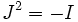# Almost complex manifold

This article defines a differential manifold with the following additional structure -- the structure group is reduced to: general linear group over complex numbers

## Definition

An almost complex manifold is the following data:

• A differential manifold$M$
• At each point, a complex structure to the vector space at that point. In other words, for every point, we give a linear map$J$ such that$J^2 = -I$, with$J$ varying smoothly with the point.